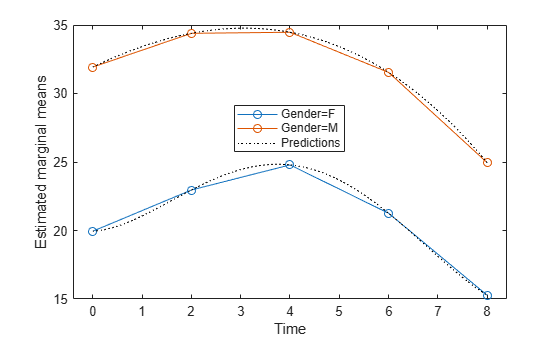# predict

Class: RepeatedMeasuresModel

Compute predicted values given predictor values

## Syntax

``ypred = predict(rm,tnew)``
``ypred = predict(rm,tnew,Name,Value)``
``````[ypred,yci] = predict(___)``````

## Description

example

````ypred = predict(rm,tnew)` returns the predicted values from the repeated measures model `rm` using the predictor values from the table `t`.```

example

````ypred = predict(rm,tnew,Name,Value)` returns the predicted values from the repeated measures model `rm` with additional options specified by one or more `Name,Value` pair arguments.For example, you can specify the within-subjects design matrix.```

example

``````[ypred,yci] = predict(___)``` also returns the 95% confidence interval for the predicted values.```

## Input Arguments

expand all

Repeated measures model, returned as a `RepeatedMeasuresModel` object.

For properties and methods of this object, see `RepeatedMeasuresModel`.

New data including the values of the response variables and the between-subject factors used as predictors in the repeated measures model, `rm`, specified as a table. `tnew` must contain all of the between-subject factors used to create `rm`.

### Name-Value Arguments

Specify optional pairs of arguments as `Name1=Value1,...,NameN=ValueN`, where `Name` is the argument name and `Value` is the corresponding value. Name-value arguments must appear after other arguments, but the order of the pairs does not matter.

Before R2021a, use commas to separate each name and value, and enclose `Name` in quotes.

Significance level of the confidence intervals for the predicted values, specified as the comma-separated pair consisting of `'alpha'` and a scalar value in the range of 0 to 1. The confidence level is 100*(1–`alpha`)%.

Example: `'alpha',0.01`

Data Types: `double` | `single`

Model for the within-subject factors, specified as the comma-separated pair consisting of `'WithinModel'` and one of the following:

• `'separatemeans'` — Compute a separate mean for each group.

• `'orthogonalcontrasts'` — Valid when the within-subject design consists of a single numeric factor T. This specifies a model consisting of orthogonal polynomials up to order T(r-1), where r is the number of repeated measures.

• A character vector or string scalar that defines a model specification in the within-subject factors.

Example: `'WithinModel','orthogonalcontrasts'`

Data Types: `char` | `string`

Design for within-subject factors, specified as the comma-separated pair consisting of `'WithinDesign'` and a vector, matrix, or a table. It provides the values of the within-subject factors in the same form as the `RM.WithinDesign` property.

Example: `'WithinDesign','Time'`

Data Types: `single` | `double` | `table`

## Output Arguments

expand all

Predicted values from the repeated measures model `rm`, returned as an n-by-r matrix, where n is the number of rows in `tnew` and r is the number of repeated measures in `rm`.

Confidence intervals for predicted values from the repeated measures model `rm`, returned as an n-by-r-by-2 matrix.

These are nonsimultaneous intervals for predicting the mean response at the specified predictor values. For predicted value `ypred(i,j)`, the lower limit of the interval is `yci(i,j,1)` and the upper limit is `yci(i,j,2)`.

## Examples

expand all

`load fisheriris`

The column vector, `species` consists of iris flowers of three different species: setosa, versicolor, and virginica. The double matrix `meas` consists of four types of measurements on the flowers: the length and width of sepals and petals in centimeters, respectively.

Store the data in a table array.

```t = table(species,meas(:,1),meas(:,2),meas(:,3),meas(:,4), ... 'VariableNames',{'species','meas1','meas2','meas3','meas4'}); Meas = dataset([1 2 3 4]','VarNames',{'Measurements'});```

Fit a repeated measures model, where the measurements are the responses and the species is the predictor variable.

`rm = fitrm(t,'meas1-meas4~species','WithinDesign',Meas);`

Predict responses for the three species.

`Y = predict(rm,t([1 51 101],:))`
```Y = 3×4 5.0060 3.4280 1.4620 0.2460 5.9360 2.7700 4.2600 1.3260 6.5880 2.9740 5.5520 2.0260 ```

`load longitudinalData`

The matrix `Y` contains response data for 16 individuals. The response is the blood level of a drug measured at five time points (time = 0, 2, 4, 6, and 8). Each row of `Y` corresponds to an individual, and each column corresponds to a time point. The first eight subjects are female, and the second eight subjects are male. This is simulated data.

Define a variable that stores gender information.

`Gender = ['F' 'F' 'F' 'F' 'F' 'F' 'F' 'F' 'M' 'M' 'M' 'M' 'M' 'M' 'M' 'M']';`

Store the data in a proper table array format to perform repeated measures analysis.

```t = table(Gender,Y(:,1),Y(:,2),Y(:,3),Y(:,4),Y(:,5), ... 'VariableNames',{'Gender','t0','t2','t4','t6','t8'});```

Define the within-subjects variable.

`Time = [0 2 4 6 8]';`

Fit a repeated measures model, where the blood levels are the responses and gender is the predictor variable.

`rm = fitrm(t,'t0-t8 ~ Gender','WithinDesign',Time);`

Predict the responses at intermediate times.

```time = linspace(0,8)'; Y = predict(rm,t([1 5 8 12],:), ... 'WithinModel','orthogonalcontrasts','WithinDesign',time);```

Plot the predictions along with the estimated marginal means.

```plotprofile(rm,'Time','Group',{'Gender'}) hold on; plot(time,Y,'Color','k','LineStyle',':'); legend('Gender=F','Gender=M','Predictions') hold off````load longitudinalData`

The matrix `Y` contains response data for 16 individuals. The response is the blood level of a drug measured at five time points (time = 0, 2, 4, 6, and 8). Each row of Y corresponds to an individual, and each column corresponds to a time point. The first eight subjects are female, and the second eight subjects are male. This is simulated data.

Define a variable that stores gender information.

`Gender = ['F' 'F' 'F' 'F' 'F' 'F' 'F' 'F' 'M' 'M' 'M' 'M' 'M' 'M' 'M' 'M']';`

Store the data in a proper table array format to perform repeated measures analysis.

```t = table(Gender,Y(:,1),Y(:,2),Y(:,3),Y(:,4),Y(:,5), ... 'VariableNames',{'Gender','t0','t2','t4','t6','t8'});```

Define the within-subjects variable.

`Time = [0 2 4 6 8]';`

Fit a repeated measures model, where the blood levels are the responses and gender is the predictor variable.

`rm = fitrm(t,'t0-t8 ~ Gender','WithinDesign',Time);`

Predict the responses at intermediate times.

```time = linspace(0,8)'; [ypred,ypredci] = predict(rm,t([1 5 8 12],:), ... 'WithinModel','orthogonalcontrasts','WithinDesign',time);```

Plot the predictions and the confidence intervals for predictions along with the estimated marginal means.

```p1 = plotprofile(rm,'Time','Group',{'Gender'}); hold on; p2 = plot(time,ypred,'Color','k','LineStyle',':'); p3 = plot(time,ypredci(:,:,1),'k--'); p4 = plot(time,ypredci(:,:,2),'k--'); legend([p1;p2(1);p3(1)],'Gender=F','Gender=M','Predictions','Confidence Intervals') hold off```